Courses

# Worksheet Questions - Data Handling Class 8 Notes | EduRev

## Mathematics (Maths) Class 8

Created by: Indu Gupta

## Class 8 : Worksheet Questions - Data Handling Class 8 Notes | EduRev

The document Worksheet Questions - Data Handling Class 8 Notes | EduRev is a part of the Class 8 Course Mathematics (Maths) Class 8.
All you need of Class 8 at this link: Class 8

Worksheet Questions (Part - 1)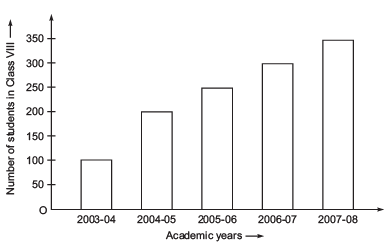(i) What is the information given by the bar graph?
(ii) In which year is the increase in the number of students is maximum?
(iii) In which year is the number of students maximum?
(iv) Is the number of students during 2005-06 twice that of 2003-04?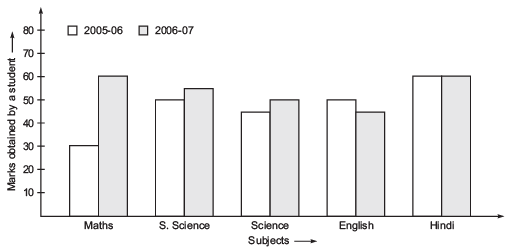(i) What is the information given by the double bar graph?
(ii) In which subject has the performance improved the most?

(iii) In which subject the performance deteriorated?
(iv) In which subject is the performance at par?

3. A group of students was asked for their favorite subject. The results were listed as under:

 Art, Mathematics, Science, English, Mathematics, Art, English, Mathematics, English, Art, Science, Art, Science, Science, Mathematics, Art, English, Art, Science, Mathematics, Science, Art.

(i) Which is the most liked subject?
(ii) Which is the least liked subject?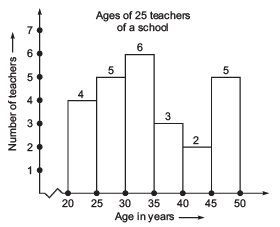(i) How many teachers are of age 45 years or more but less than 50 years?
(ii) How many teachers are of age less than 35 years?

1. (i) Number of students in class VIII in different academic years.
(ii) 2004-05
(iii) 2007-08
(iv) No

2. (i) Marks obtained by a student in different subjects by a student in 2005-06 and 2006-07.
(ii) Mathematics
(iii) English
(iv) Hindi

3. (i) Science
(ii) English

4. (i) 5 teachers
(ii) 4 + 5 + 6 = 15 teachers

Worksheet Questions (Part - 2)
1. Read the following circle graphs and answer the questions given below:

(a) The time spent by a child during a day:
(i) On which activity maximum number of hours are spent?
(ii) On which two activities does he spend equal number of hours?
(iii) Find the central angles for each sector of activities.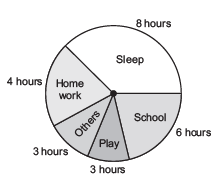(b) Age group of people in a town:
(i) In which age group are the maximum number of people?
(ii) How many people are there is the ‘0-14 years’ group?
(iii) Find the central angle of the sector corresponding to the age group ’15-60 years’.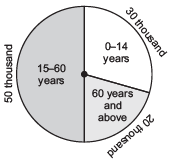3. The favourate flavours of ice-creams for students of a school is given in percentages as follows:

 Flavours Percentage of students Preferring the flavours Chocolate 50% Vanilla 25% Other flavours 25%

Represent the data in a pie chart.

4. The adjoining pie chart gives the expenditure (in percentages) on various items and savings of a family during a month.
(i) On which item, the expenditure was minimum?
(ii) Expenditure on which item is equal to the total saving of the family?
(iii) If the monthly saving of the family is Rs 3000, then what is the monthly expenditure on clothes?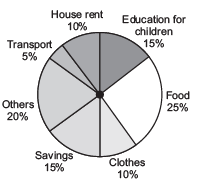5. On a particular day, the sales (in rupees) of various items of baker’s shop are given below. Draw a pie chart for this data:

 ordinary bread 320 fruit bread 80 biscuits 120 cakes and pastries 160 others 40 Total 720

1. (i) Sleep
(ii) Play and others
(iii) Sleep 120°, school 90°, how work 60°; play 45°, others 45°

2. (i) 15–60 years
(ii) 30 thousand
(iii) 180°

4. (i) Transport
(ii) Education for children
(iii) Rs 2000

Worksheet Questions (Part - 3)
1. List the possible outcomes in the adjoining spinning wheel.

2. (i) A die is thrown. List the possible outcomes.
(ii) What is probability of getting a factor of 6?

Hint: Factors of six are 1, 2, 3 and 6.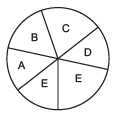(iii) What is the probability of getting a number greater than 6?

Note: A die does not have a number greater than 6 on its face. Therefore, the possible outcomes are zero.

3. (i) Find the number of outcomes of getting a sector containing G.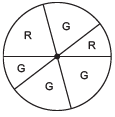(ii) What is the probability of getting a sector containing R?

4. A bag has 4-red balls and 2 yellow balls such that the balls are identical in all repeats other than colour. A ball is drawn from the bag without looking into the bag. What is the probability of getting yellow ball?

1. A, B, C, D, E

2. (i) 1, 2, 3, 4, 5, 6
(ii) 2/3
(iii) 0

3. 4
4. 1/3

,

,

,

,

,

,

,

,

,

,

,

,

,

,

,

,

,

,

,

,

,

;